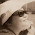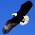Wednesday, 30 January 2013

20130130 Task 1: Do you agree with Juliet's conclusion?

Juliet attempts to explain that square root and cube root of numbers can be rational numbers. She gave several examples to illustrate her points. Do you fully agree with her?
• If you do, support your response with more illustrations to show that it is always true.
• If you do not, give examples to illustrate why you do not agree.
Enter your response to the following in "Comments".

1.Not true.Rational numbers can be expressed as a/b where b is not equal to 0.the square root of 2 doesn't give a rational number as it cannot be expresses in a/b and thus not all square root and cube root of any numbers or fractions will result a rational number.

1.Taufiq:
You only gave a counter-example for square root, i.e. Square Root of 2.
How about Cube Root? Need to elaborate too :)

2.It applies the same thing,When i cube root e.g.7,I can't express the answer as a/b,so its not a rational number

2.It is not true. Rational numbers must be able to be expressed in x/y while y is not 0. The square root of some numbers eg. 2 cannot be expressed in x/y and the cube root of some numbers eg. 5 cannot be expressed in x/y

1.3.Rational numbers are numbers that can be express as a/b and b cannot be 0.2 when square-rooted,becomes a number that cannot be expressed as a/b. Numbers like 4 cannot be cube rooted.

1.4.RAtional numbers are numbers that can be in the form of a/b and b must not be a zero.Numbers like 2 cannot be square rooted into a fraction and numbers like 5 cannot be cuberooted into a factions, thus I do not agree with her

5.Not true. Rational numbers are numbers that can be shown as x/y and y can not be 0. Henceforth, numbers like 5 cannot be square rooted into a fraction and it also can't be cube rooted into a rational number. So I do not agree

6.It is not true. Rational numbers are numbers that can be expressed as a/b where b is not 0. The square root of 3 does not give a rational number.The cube of root of 5 is also not a rational number because it cannot be expressed in a/b.

7.It is not true as rational numbers are whole numbers and fractions,in which b does not equal to 0 when it is expressed as a/b. However, not all square root and cube root are rational numbers as for example the square root of 5 and the cube root of 6 are irrational numbers but they are still square root and cube root.

8.It is not true because a rational number is a/b where a and b are integers and b is not a zero. for example the square root of 2 is 1.4142... and 1.4142... is impossible to be expressed as such where the rules apply. for example the cube root of 10 is 2.1544... and 2.1544 cannot be expressed as such where the rules apply. Therefore Juliet's statement is wrong

9.This is not true as some numbers cannot be cube rooted or square rooted as the answer would have a lot of numbers after the decimal, therefore not being able to be converted to a fraction. Hence they may not be rational numbers.

10.Only the perfect squares and cubes give a rational number. Others give irrational numbers.

11.Not true. For e.g. square root of 2 cannot and the cube root of two cannot be expressed as a fraction a/b, with b not being zero, thus not all square root or cube root of any numbers will result in a rational number and JULIET'S STATEMENT IS WRONG. YAY!!!:D

12.no, its not true as rational numbers can be expressed by a/b where b is not 0.The square root of 3 is not a rational number and the cube root of 5 is also not a rational number as it also cannot be expressed in a/b thus i do not agree with juliet's statement.

13.This is not true as some numbers when square rooted and cube rooted will result in 'infinite' decimals,examples are square root of 2,square root of 3,cube root of 2 and cube root of 3.Since these numbers have 'infinite' decimals,we cannot convert them to fractions,hence not resulting to be rational numbers.

14.It is not true. Rational numbers are numbers that can be expressed as a fraction as long as the denominator is not 0 . But The square root of 2 does not give us a rational number.The following also applies fot the cube root. The cube root of 7 does not give us a rational number

15.Not true. Rational numbers can be whole and natural numbers or expressed as a fraction (a/b), but square root of 2, 3, 5 and others may not result in a whole number or a/b. Same for cube root. 2, 4, 5 and others cannot be expressed in such manner, therefore the whol statement is wrong.

16.False. Rational numbers are numbers that can be expressed as a fraction without 0 as the denominator. But some numbers like square root of 2, cube root of 4 will result in reoccurring decimals, which are irrational numbers.

17.It is not true. Rational numbers must be expressed as a fraction (a/b form where b is not equal to 0). When we square root 6, we get a decimal which cannot be expressed as a fraction. It is the same for cube root, for eg, if we cube root 10, we also get a decimal which cannot be expressed as a fraction. (They are all irrational) So this means that square root or cube root of any numbers or fractions will not result in a rational number.

18.Not true. Rational numbers are numbers that can be expressed as fraction e.g. a/b where b must not equal to 0. When we square root or cube root 7 we will not get a rational number.

19.It is not true.Rational numbers are numbers that can be expressed as a fraction in the form a/b and b is not 0. When we square root 2, it cannot be expressed into fraction. When we cube root 10, it also cannot be expressed in a fraction.

20.It is not true. Rational numbers are numbers that are expressed as a fraction in the form of a/b but b cannot be zero. When we square root the number 2, it cannot be expressed into a fraction. Same goes with 7 and 10